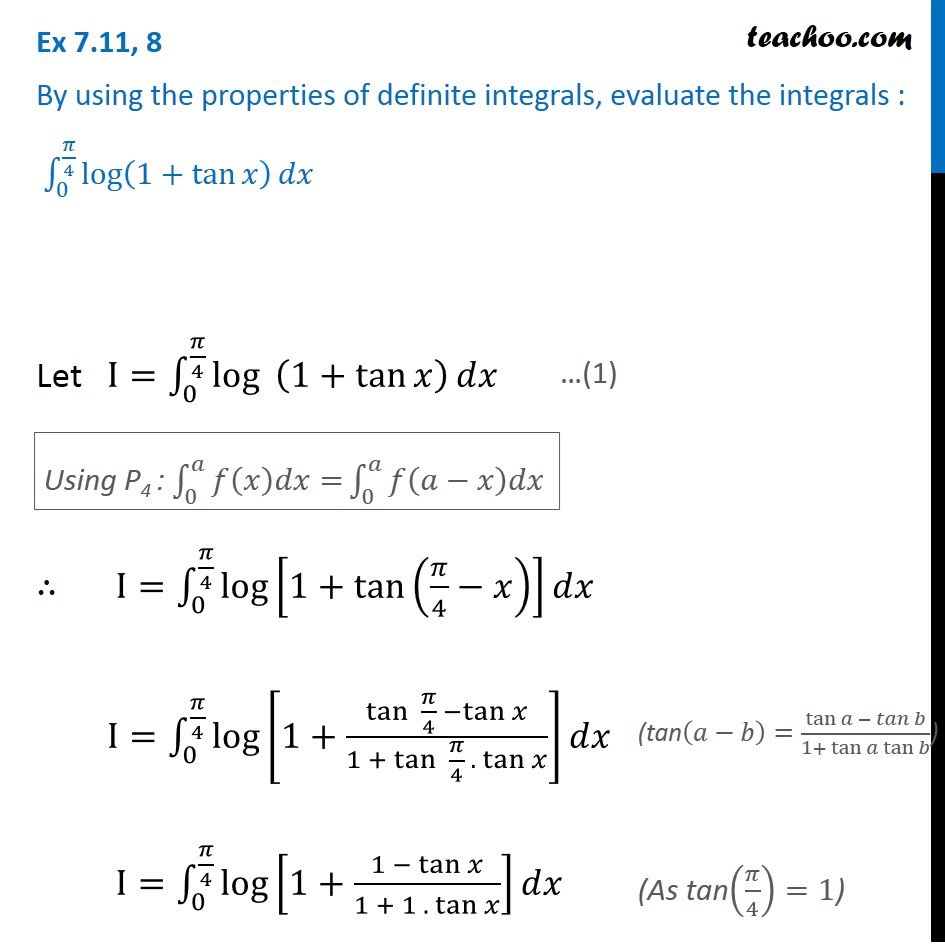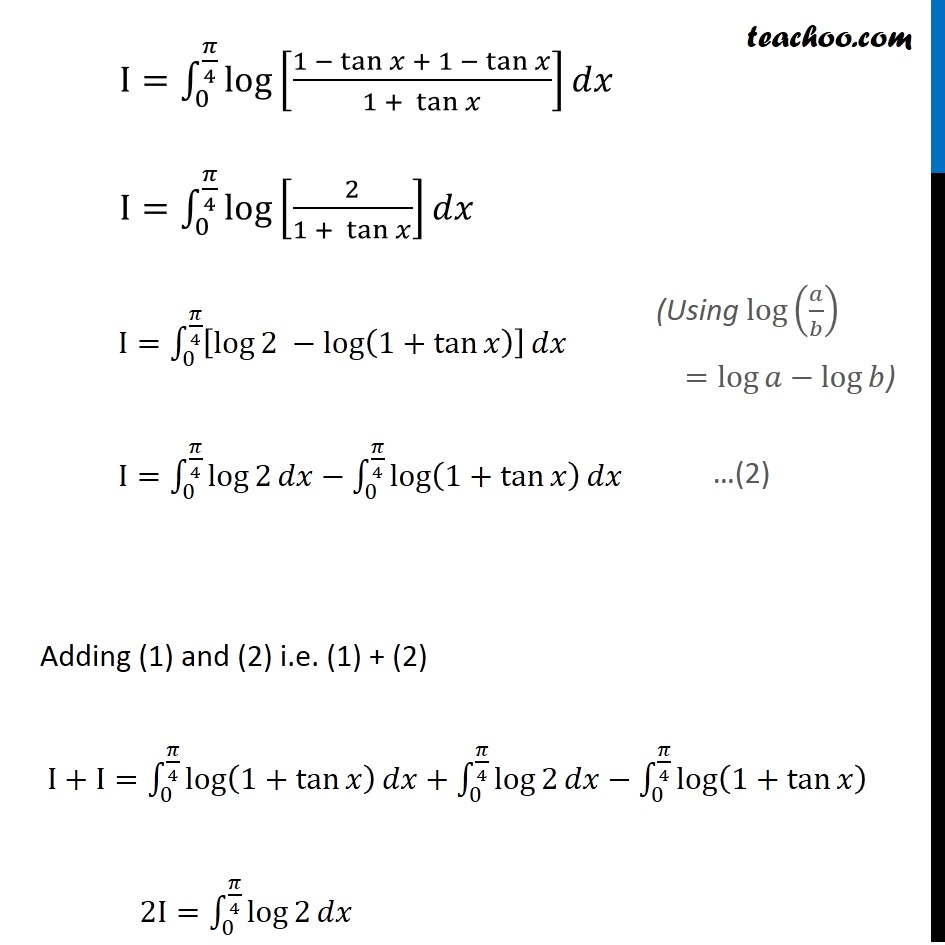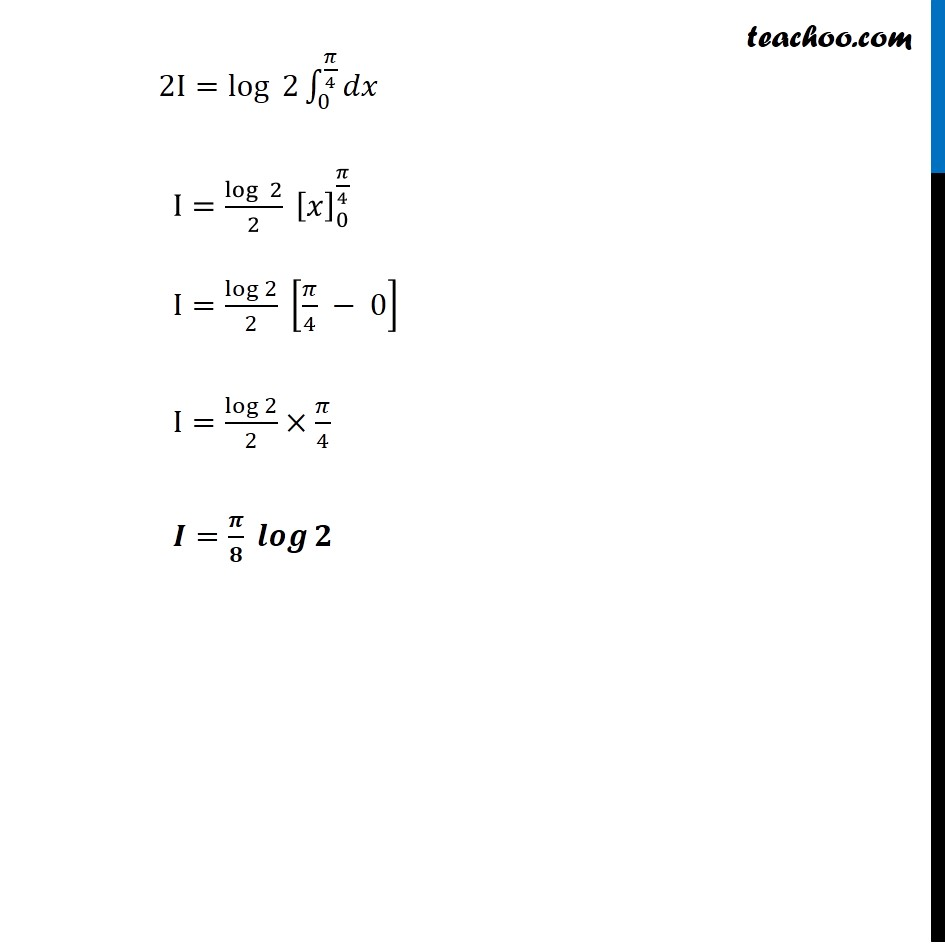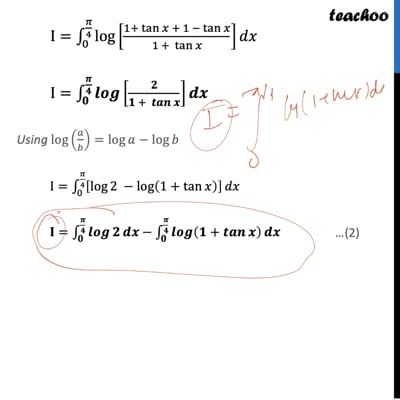Ex 7.11

Chapter 7 Class 12 Integrals
Serial order wiseThis video is only available for Teachoo black users

Get live Maths 1-on-1 Classs - Class 6 to 12

### Transcript

Ex 7.11, 8 By using the properties of definite integrals, evaluate the integrals : ∫_0^(𝜋/4)▒log⁡(1+tan⁡𝑥 ) 𝑑𝑥 Let I=∫_0^(𝜋/4)▒log⁡〖 (1+tan⁡𝑥 )〗 𝑑𝑥 ∴ I=∫_0^(𝜋/4)▒log⁡[1+tan⁡(𝜋/4−𝑥) ] 𝑑𝑥 I=∫_0^(𝜋/4)▒log⁡[1+(tan⁡ 𝜋/4 −tan⁡𝑥)/(1 +〖 tan〗⁡ 𝜋/4 . tan⁡𝑥 )] 𝑑𝑥 I=∫_0^(𝜋/4)▒log⁡[1+(1 − tan⁡𝑥)/(1 + 1 . tan⁡𝑥 )] 𝑑𝑥 Ex 7.11, 8 By using the properties of definite integrals, evaluate the integrals : ∫_0^(𝜋/4)▒log⁡(1+tan⁡𝑥 ) 𝑑𝑥 Let I=∫_0^(𝜋/4)▒log⁡〖 (1+tan⁡𝑥 )〗 𝑑𝑥 ∴ I=∫_0^(𝜋/4)▒log⁡[1+tan⁡(𝜋/4−𝑥) ] 𝑑𝑥 I=∫_0^(𝜋/4)▒log⁡[1+(tan⁡ 𝜋/4 −tan⁡𝑥)/(1 +〖 tan〗⁡ 𝜋/4 . tan⁡𝑥 )] 𝑑𝑥 I=∫_0^(𝜋/4)▒log⁡[1+(1 − tan⁡𝑥)/(1 + 1 . tan⁡𝑥 )] 𝑑𝑥 (tan(𝑎−𝑏)=tan⁡〖𝑎 − 𝑡𝑎𝑛 𝑏〗/(1+〖 tan〗⁡〖𝑎 tan⁡𝑏 〗 )) (As tan(𝜋/4)=1) I=∫_0^(𝜋/4)▒log⁡[(1 − tan⁡𝑥 + 1 − tan⁡𝑥)/(1 + tan⁡𝑥 )] 𝑑𝑥 I=∫_0^(𝜋/4)▒log⁡[2/(1 + tan⁡𝑥 )] 𝑑𝑥 I=∫_0^(𝜋/4)▒[log⁡2 −log⁡(1+tan⁡𝑥 ) ] 𝑑𝑥 I=∫_0^(𝜋/4)▒log⁡2 𝑑𝑥−∫_0^(𝜋/4)▒log⁡(1+tan⁡𝑥 ) 𝑑𝑥 Adding (1) and (2) i.e. (1) + (2) I+I=∫_0^(𝜋/4)▒log⁡(1+tan⁡𝑥 ) 𝑑𝑥+∫_0^(𝜋/4)▒log⁡2 𝑑𝑥−∫_0^(𝜋/4)▒log⁡(1+tan⁡𝑥 ) 2I=∫_0^(𝜋/4)▒log⁡2 𝑑𝑥 (Using log⁡(𝑎/𝑏) =log⁡𝑎−log⁡𝑏) …(2) 2I=log⁡〖 2〗 ∫_0^(𝜋/4)▒𝑑𝑥 I=log⁡〖 2〗/2 [𝑥]_0^(𝜋/4) I=log⁡2/2 [𝜋/4 − 0] I=log⁡2/2×𝜋/4 𝑰=𝝅/𝟖 𝒍𝒐𝒈⁡𝟐